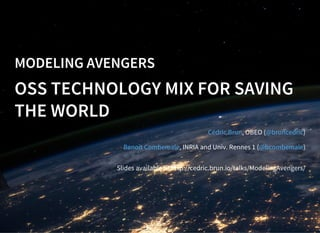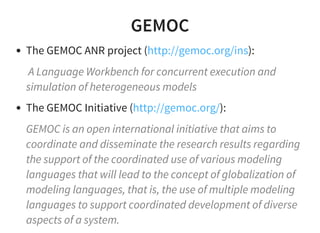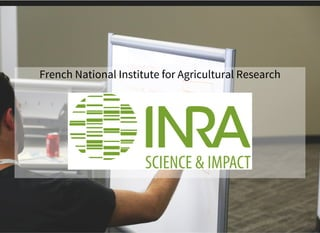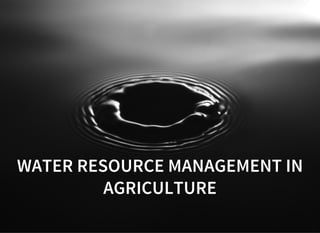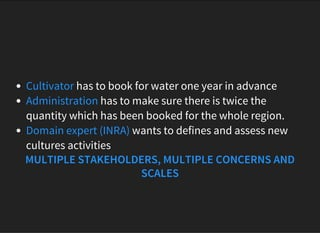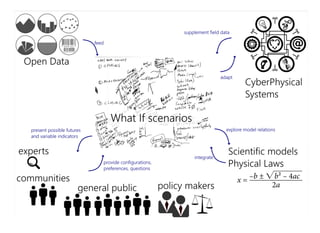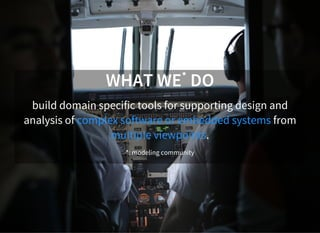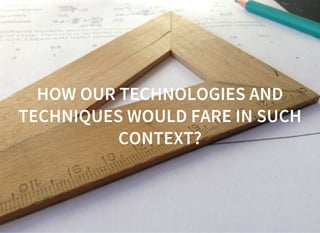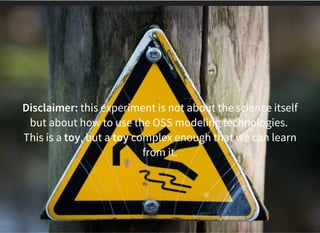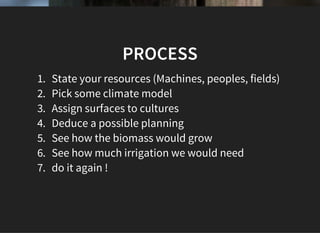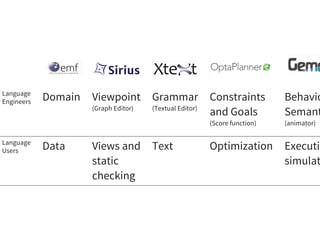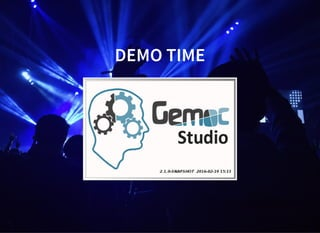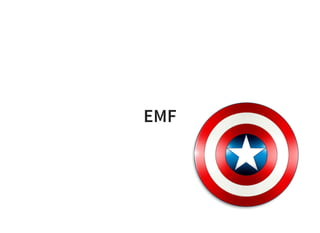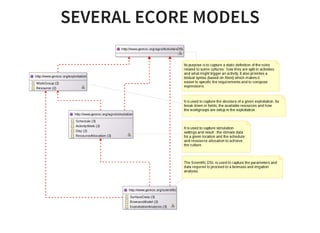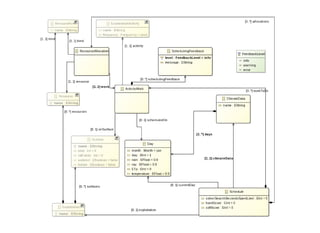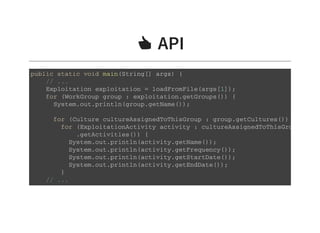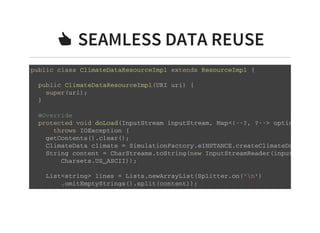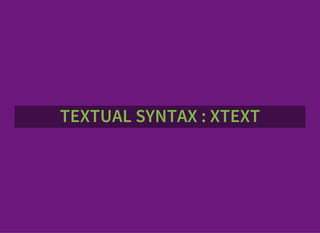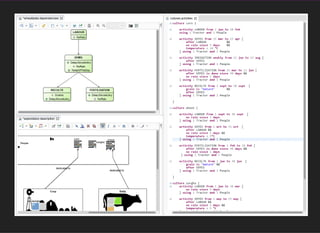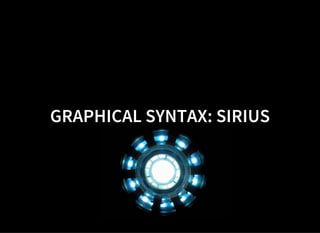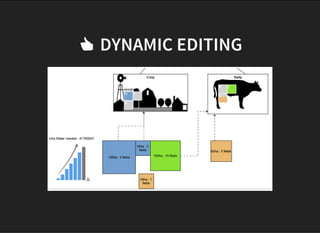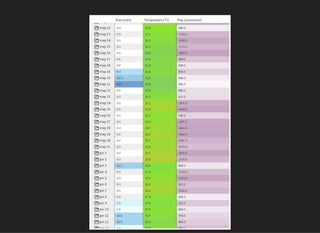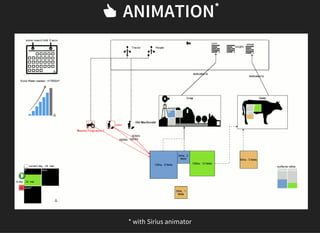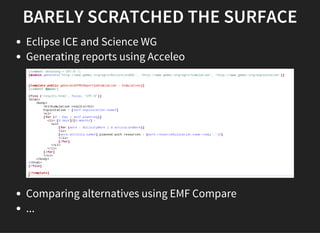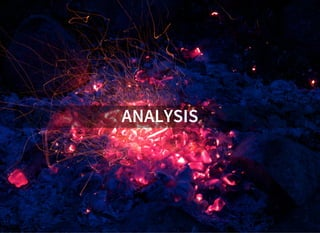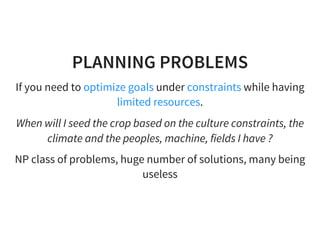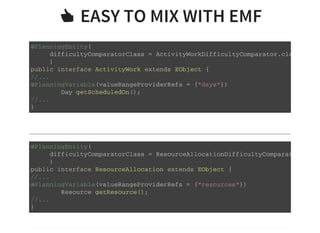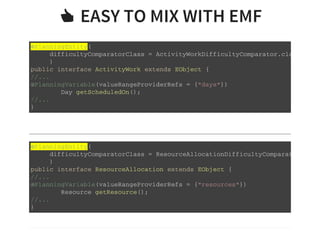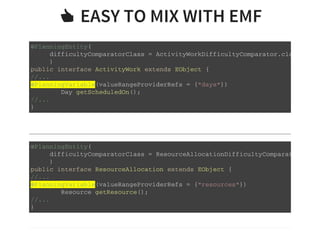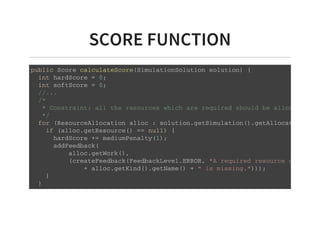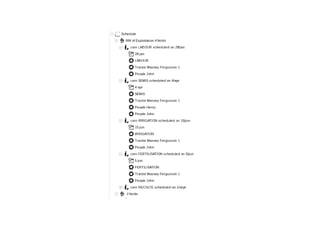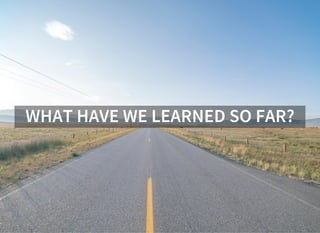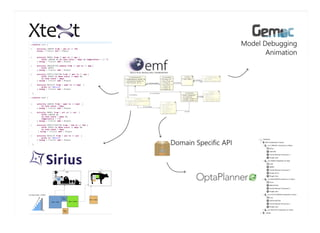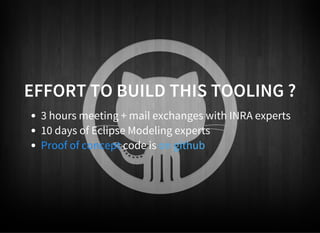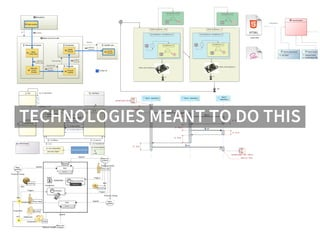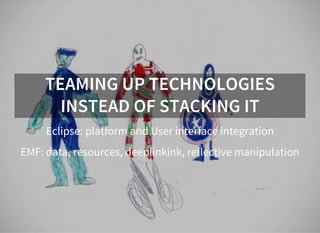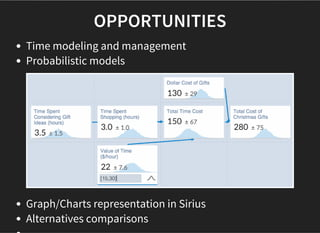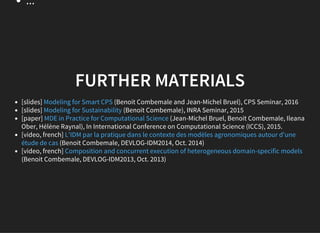1 of 44

### Modeling avengers – open source technology mix for saving the world

1. MODELING AVENGERS OSS TECHNOLOGY MIX FOR SAVING THE WORLD , OBEO ( ) , INRIA and Univ. Rennes 1 ( ) Cédric Brun @bruncedric Benoit Combemale @bcombemale Slides available at http://cedric.brun.io/talks/ModelingAvengers/
2. GEMOC The GEMOC ANR project ( ): A Language Workbench for concurrent execution and simulation of heterogeneous models The GEMOC Initiative ( ): GEMOC is an open international initiative that aims to coordinate and disseminate the research results regarding the support of the coordinated use of various modeling languages that will lead to the concept of globalization of modeling languages, that is, the use of multiple modeling languages to support coordinated development of diverse aspects of a system. http://gemoc.org/ins http://gemoc.org/
3. French National Institute for Agricultural Research
4.       WATER RESOURCE MANAGEMENT IN AGRICULTURE
5. Cultivator has to book for water one year in advance Administration has to make sure there is twice the quantity which has been booked for the whole region. Domain expert (INRA) wants to defines and assess new cultures activities MULTIPLE STAKEHOLDERS, MULTIPLE CONCERNS AND SCALES
6. CULTIVATOR Which field to use for growing what ? How would the crops grows? When would I have to add water ? Machines ? Peoples ? COMPLEX SYSTEM, OPTIMIZATION WITH MULTIPLE FACTORS (WEATHER, COSTS, RESOURCES)
7. WHAT WE* DO build domain specific tools for supporting design and analysis of complex software or embedded systems from multiple viewpoints. *: modeling community
8. HOW OUR TECHNOLOGIES AND TECHNIQUES WOULD FARE IN SUCH CONTEXT?
9. Disclaimer: this experiment is not about the science itself but about how to use the OSS modeling technologies. This is a toy, but a toy complex enough that we can learn from it.
10. PROCESS 1. State your resources (Machines, peoples, fields) 2. Pick some climate model 3. Assign surfaces to cultures 4. Deduce a possible planning 5. See how the biomass would grow 6. See how much irrigation we would need 7. do it again !
11. Language Engineers Domain Viewpoint (Graph Editor) Grammar (Textual Editor) Constraints and Goals (Score function) Behavio Semant (animator) Language Users Data Views and static checking Text Optimization Executio simulat
12. DEMO TIME
13. EMF
14. SEVERAL ECORE MODELS
15. ὄ API             p u b l i c s t a t i c v o i d m a i n ( S t r i n g [ ] a r g s ) { / / . . . E x p l o i t a t i o n e x p l o i t a t i o n = l o a d F r o m F i l e ( a r g s [ 1 ] ) ; f o r ( W o r k G r o u p g r o u p : e x p l o i t a t i o n . g e t G r o u p s ( ) ) { S y s t e m . o u t . p r i n t l n ( g r o u p . g e t N a m e ( ) ) ; f o r ( C u l t u r e c u l t u r e A s s i g n e d T o T h i s G r o u p : g r o u p . g e t C u l t u r e s ( ) ) { f o r ( E x p l o i t a t i o n A c t i v i t y a c t i v i t y : c u l t u r e A s s i g n e d T o T h i s G r o u p . g e t A c t i v i t i e s ( ) ) { S y s t e m . o u t . p r i n t l n ( a c t i v i t y . g e t N a m e ( ) ) ; S y s t e m . o u t . p r i n t l n ( a c t i v i t y . g e t F r e q u e n c y ( ) ) ; S y s t e m . o u t . p r i n t l n ( a c t i v i t y . g e t S t a r t D a t e ( ) ) ; S y s t e m . o u t . p r i n t l n ( a c t i v i t y . g e t E n d D a t e ( ) ) ; } / / . . .
16. ὄ SEAMLESS DATA REUSE             p u b l i c c l a s s C l i m a t e D a t a R e s o u r c e I m p l e x t e n d s R e s o u r c e I m p l { p u b l i c C l i m a t e D a t a R e s o u r c e I m p l ( U R I u r i ) { s u p e r ( u r i ) ; } @ O v e r r i d e p r o t e c t e d v o i d d o L o a d ( I n p u t S t r e a m i n p u t S t r e a m , M a p < ! - - ? , ? - - > o p t i o n s ) t h r o w s I O E x c e p t i o n { g e t C o n t e n t s ( ) . c l e a r ( ) ; C l i m a t e D a t a c l i m a t e = S i m u l a t i o n F a c t o r y . e I N S T A N C E . c r e a t e C l i m a t e D a t a ( ) ; S t r i n g c o n t e n t = C h a r S t r e a m s . t o S t r i n g ( n e w I n p u t S t r e a m R e a d e r ( i n p u t S t r e a m , C h a r s e t s . U S _ A S C I I ) ) ; L i s t < s t r i n g > l i n e s = L i s t s . n e w A r r a y L i s t ( S p l i t t e r . o n ( ' n ' ) . o m i t E m p t y S t r i n g s ( ) . s p l i t ( c o n t e n t ) ) ;
17. TEXTUAL SYNTAX : XTEXT
18. ὄ PREDICATES, EXPRESSIONS, CONDITIONS
19. GRAPHICAL SYNTAX: SIRIUS
20. ὄ DYNAMIC EDITING
21. ὄ TABLE EDITORS
22. ὄ ANIMATION* * with Sirius animator
23. BARELY SCRATCHED THE SURFACE Eclipse ICE and Science WG Generating reports using Acceleo Comparing alternatives using EMF Compare ...
24. ANALYSIS
25. PLANNING PROBLEMS If you need to optimize goals under constraints while having limited resources. When will I seed the crop based on the culture constraints, the climate and the peoples, machine, fields I have ? NP class of problems, huge number of solutions, many being useless
26. ὄ EASY TO MIX WITH EMF             @ P l a n n i n g E n t i t y ( d i f f i c u l t y C o m p a r a t o r C l a s s = A c t i v i t y W o r k D i f f i c u l t y C o m p a r a t o r . c l a s s ) p u b l i c i n t e r f a c e A c t i v i t y W o r k e x t e n d s E O b j e c t { / / . . . @ P l a n n i n g V a r i a b l e ( v a l u e R a n g e P r o v i d e r R e f s = { " d a y s " } ) D a y g e t S c h e d u l e d O n ( ) ; / / . . . }                       @ P l a n n i n g E n t i t y ( d i f f i c u l t y C o m p a r a t o r C l a s s = R e s o u r c e A l l o c a t i o n D i f f i c u l t y C o m p a r a t o r . c l a s s ) p u b l i c i n t e r f a c e R e s o u r c e A l l o c a t i o n e x t e n d s E O b j e c t { / / . . . @ P l a n n i n g V a r i a b l e ( v a l u e R a n g e P r o v i d e r R e f s = { " r e s o u r c e s " } ) R e s o u r c e g e t R e s o u r c e ( ) ; / / . . . }
27. ὄ EASY TO MIX WITH EMF             @ P l a n n i n g E n t i t y ( d i f f i c u l t y C o m p a r a t o r C l a s s = A c t i v i t y W o r k D i f f i c u l t y C o m p a r a t o r . c l a s s ) p u b l i c i n t e r f a c e A c t i v i t y W o r k e x t e n d s E O b j e c t { / / . . . @ P l a n n i n g V a r i a b l e ( v a l u e R a n g e P r o v i d e r R e f s = { " d a y s " } ) D a y g e t S c h e d u l e d O n ( ) ; / / . . . }                       @ P l a n n i n g E n t i t y ( d i f f i c u l t y C o m p a r a t o r C l a s s = R e s o u r c e A l l o c a t i o n D i f f i c u l t y C o m p a r a t o r . c l a s s ) p u b l i c i n t e r f a c e R e s o u r c e A l l o c a t i o n e x t e n d s E O b j e c t { / / . . . @ P l a n n i n g V a r i a b l e ( v a l u e R a n g e P r o v i d e r R e f s = { " r e s o u r c e s " } ) R e s o u r c e g e t R e s o u r c e ( ) ; / / . . . }
28. ὄ EASY TO MIX WITH EMF             @ P l a n n i n g E n t i t y ( d i f f i c u l t y C o m p a r a t o r C l a s s = A c t i v i t y W o r k D i f f i c u l t y C o m p a r a t o r . c l a s s ) p u b l i c i n t e r f a c e A c t i v i t y W o r k e x t e n d s E O b j e c t { / / . . . @ P l a n n i n g V a r i a b l e ( v a l u e R a n g e P r o v i d e r R e f s = { " d a y s " } ) D a y g e t S c h e d u l e d O n ( ) ; / / . . . }                       @ P l a n n i n g E n t i t y ( d i f f i c u l t y C o m p a r a t o r C l a s s = R e s o u r c e A l l o c a t i o n D i f f i c u l t y C o m p a r a t o r . c l a s s ) p u b l i c i n t e r f a c e R e s o u r c e A l l o c a t i o n e x t e n d s E O b j e c t { / / . . . @ P l a n n i n g V a r i a b l e ( v a l u e R a n g e P r o v i d e r R e f s = { " r e s o u r c e s " } ) R e s o u r c e g e t R e s o u r c e ( ) ; / / . . . }
29. SCORE FUNCTION             p u b l i c S c o r e c a l c u l a t e S c o r e ( S i m u l a t i o n S o l u t i o n s o l u t i o n ) { i n t h a r d S c o r e = 0 ; i n t s o f t S c o r e = 0 ; / / . . . / * * C o n s t r a i n t : a l l t h e r e s o u r c e s w h i c h a r e r e q u i r e d s h o u l d b e a l l o c a t e d . * / f o r ( R e s o u r c e A l l o c a t i o n a l l o c : s o l u t i o n . g e t S i m u l a t i o n ( ) . g e t A l l o c a t i o n s ( ) ) i f ( a l l o c . g e t R e s o u r c e ( ) = = n u l l ) { h a r d S c o r e + = m e d i u m P e n a l t y ( 1 ) ; a d d F e e d b a c k ( a l l o c . g e t W o r k ( ) , ( c r e a t e F e e d b a c k ( F e e d b a c k L e v e l . E R R O R , " A r e q u i r e d r e s o u r c e o f k i n d " + a l l o c . g e t K i n d ( ) . g e t N a m e ( ) + " i s m i s s i n g . " ) ) ) ; } }
30. WHAT HAVE WE LEARNED SO FAR?
31. “Do not compromise on your domain model.”
32. EFFORT TO BUILD THIS TOOLING ? 3 hours meeting + mail exchanges with INRA experts 10 days of Eclipse Modeling experts Proof of concept code is on github
33. TECHNOLOGIES MEANT TO DO THIS
34. ARE RELEVANT TO SAVE THIS
35. TEAMING UP TECHNOLOGIES INSTEAD OF STACKING IT Eclipse: platform and User interface integration EMF: data, resources, deeplinkink, reflective manipulation
36. OPPORTUNITIES Time modeling and management Probabilistic models Graph/Charts representation in Sirius Alternatives comparisons
37. ... FURTHER MATERIALS [slides] (Benoit Combemale and Jean-Michel Bruel), CPS Seminar, 2016 [slides] (Benoit Combemale), INRA Seminar, 2015 [paper] (Jean-Michel Bruel, Benoit Combemale, Ileana Ober, Hélène Raynal), In International Conference on Computational Science (ICCS), 2015. [video, french] (Benoit Combemale, DEVLOG-IDM2014, Oct. 2014) [video, french] (Benoit Combemale, DEVLOG-IDM2013, Oct. 2013) Modeling for Smart CPS Modeling for Sustainability MDE in Practice for Computational Science L'IDM par la pratique dans le contexte des modèles agronomiques autour d'une étude de cas Composition and concurrent execution of heterogeneous domain-specific models